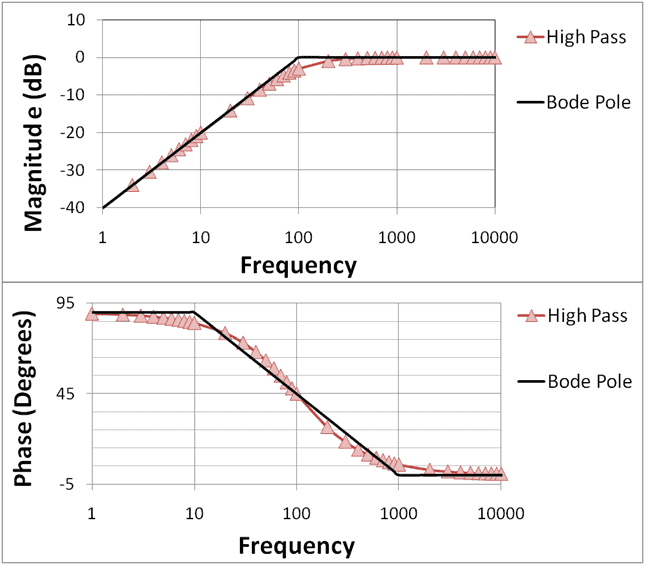# Bode Plot Application: Increase Frequency Response & Magnitude Understanding

• SDRFG
In summary, you are looking for a function that will describe the magnitude and phase response of a simple frequency-dependent network. You have found this information in a wiki article, but you need to understand the meaning of "s" and how to calculate magnitude and phase. Once you have these expressions, you can return and ask more specific questions.

#### SDRFG

Hello,
After long time i am able to find Laplace transform function but can't understand the bode plot which give information of frequency response and magnitude i think
plaese anyway here how can push my thinking ahead in it?

https://en.m.wikipedia.org/wiki/Bode_plot

You can't get a whole semester course from an online forum. I suggest that you try hard to understand the wiki article linked. After that, you can return here with more specific questions and we will try to help.

•SDRFG
ok, there is function s+1/s+2

how to draw bode plot?
You can't get a whole semester course
i was not able to understand at that time.Should both diagrams be correlated?

•SDRFG
no because one show for phase and another show for magnitude.

SDRFG said:
no because one show for phase and another show for magnitude.
Sorry - but this is not an answer to my question (I did not ask if both are "equal").
The complete BODE diagram for each frequency dependent network consists of TWO drawings: (a) Magnitude vs. frequency and (b) phase vs. frequency.
You have shown two such diagrams - however, it seems that they are not for the same circuit (because the must be correlated!).

•SDRFG
i have no idea get it from wiki.
any way how they should be correlated..

As I wrote already: Each frequency-dependent circuit (most simple example: RC lowpass) can be split into a magnitude and a phase function.
That is pure mathematics: H(jω)=|H(ω)|*exp(φ(ω)).
So you have two functions which are, of course, correlated because they come from the same circuit.

•SDRFG
how to learn bode plot??

SDRFG said:
how to learn bode plot??
Simple answer: Learn how to produce a graphical representation of magnitude and phase of a simple frequency-dependent network (R-C combination).
Start with H(jw)=Vout/Vin and calculate magnitude and phase functions. This requires some basic math knowledge.

•SDRFG
Start with H(jw)=Vout/Vin and calculate magnitude and phase functions. This requires some basic math knowledge.
i have got Vo/Vi=s+1/s+2

SDRFG said:
i have got Vo/Vi=s+1/s+2
I suppose you mean (s+1)/(s+2), correct?
* Do you know the meaning of "s"?
* Are you able to write down the expressions for magnitude and phase?

after that i dnt not no please teach me after what is s and s=jw may be zero poles
no i frget i will remijnd if you follow